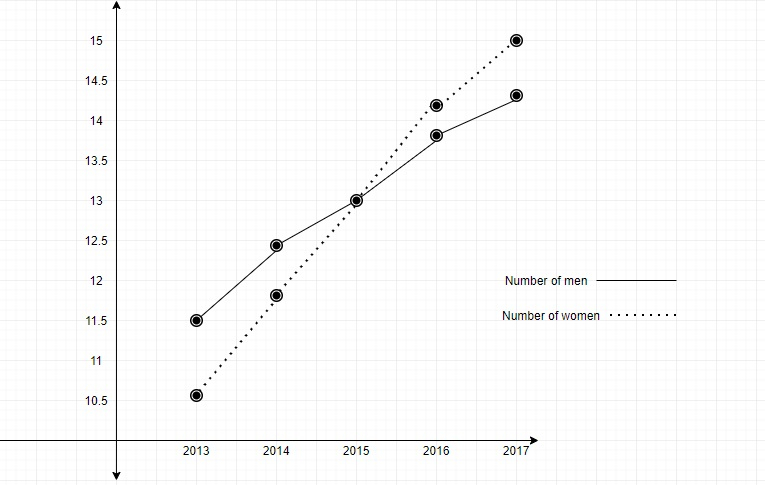Year20132014201520162017Number of men11.512.41313.814.2Number of women10.511.71314.215.0Draw two line graphs for the above data">

# The table given below shows the population (in thousands) of men and women in a village in different years.Year20132014201520162017Number of men11.512.41313.814.2Number of women10.511.71314.215.0Draw two line graphs for the above data

Let us take the years on x-axis and population on the y-axis.

Taking the scale 1 unit $=$ population of 500 on the y-axis and 1 unit $=$ 2 years on the x-axis.(i) The ratio of the male population and female population in the year $2013=\frac{11.5\times1000}{10.5\times1000}=\frac{115}{105}=\frac{23}{21}$.

(ii) The ratio of the total population of the village in 2015 to that in 2017 $=\frac{(13+13)\times1000}{(14.2+15)\times1000}=\frac{26}{29.2}=\frac{130}{146}=\frac{65}{73}$

(iii)  The percentage increase in the population of women in 2017 as compared to that in 2014$=\frac{(15-11.7)\times1000}{11.7\times1000}\times100 \%=\frac{33}{117}\times100 \%=\frac{11}{39}\times100 \%=28.21 \%$

(iv) Percentage increase in the men from 2013 to 2014$=\frac{(12.4-11.5)\times1000}{11.5\times1000}\times100 \%=\frac{9}{115}\times100 \%=\frac{900}{115} \%=7.82 \%$

Percentage increase in the men from 2014 to 2015$=\frac{(13-12.4)\times1000}{12.4\times1000}\times100 \%=\frac{6}{124}\times100 \%=\frac{600}{124} \%=4.84 \%$

Percentage increase in the men from 2015 to 2016$=\frac{(13.8-13)\times1000}{13\times1000}\times100 \%=\frac{8}{130}\times100 \%=\frac{80}{13} \%=6.15 \%$

Percentage increase in the men from 2016 to 2017$=\frac{(14.2-13.8)\times1000}{13.8\times1000}\times100 \%=\frac{4}{138}\times100 \%=\frac{400}{138} \%=2.90 \%$

Between 2013 and 2014 the percentage increase in the number of men is maximum.

Updated on: 10-Oct-2022

96 Views